(page 2 of 4)

Sections: Introduction, The meaning of the leading coefficient / The vertex, ExamplesThe general form of a quadratic is "y = ax2 + bx + c". For graphing, the leading coefficient "a" indicates how "fat" or how "skinny" the parabola will be.

For | a | > 1 (such as a = 3 or a = –4), the parabola will be "skinny", because it grows more quickly (three times as fast or four times as fast, respectively, in the case of our sample values
of
a).

For | a | < 1 (such as a = 1/3 or a = –1/4 ), the parabola will be "fat", because it grows more slowly (one-third as fast or one-fourth as fast, respectively, in the examples). Also, if a is negative, then the parabola is upside-down.

 You can see these trends when you look at how the curve y = ax2 moves as "a" changes: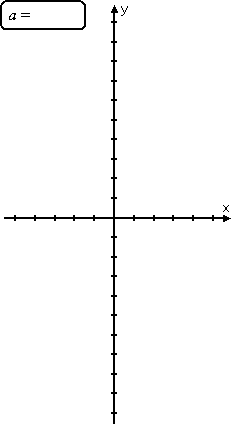As you can see, as the leading coefficient goes from very negative to slightly negative to zero (not really a quadratic) to slightly positive to very positive, the parabola goes from skinny upside-down to fat upside-down to a straight line (called a "degenerate" parabola) to a fat right-side-up to a skinny right-side-up.   Copyright © Elizabeth Stapel 2002-2011 All Rights Reserved

There is a simple, if slightly "dumb", way to remember the difference between right-side-up parabolas and upside-down parabolas:

 positive quadratic y = x2 negative quadratic y = –x2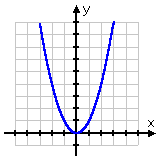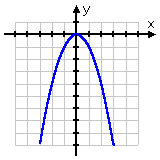This can be useful information: If, for instance, you have an equation where a is negative, but you're somehow coming up with plot points that make it look like the quadratic is right-side-up, then you will know that you need to go back and check your work, because something is wrong.

Parabolas always have a lowest point (or a highest point, if the parabola is upside-down). This point, where the parabola changes direction, is called the "vertex".

If the quadratic is written in the form y = a(xh)2 + k, then the vertex is the point (h, k). This makes sense, if you think about it. The squared part is always positive (for a right-side-up parabola), unless it's zero. So you'll always have that fixed value k, and then you'll always be adding something to it to make y bigger, unless of course the squared part is zero. So the smallest y can possibly be is y = k, and this smallest value will happen when the squared part, xh, equals zero. And the squared part is zero when xh = 0, or when x = h. The same reasoning works, with k being the largest value and the squared part always subtracting from it, for upside-down parabolas.

(Note: The "a" in the vertex form "y = a(xh)2 + k" of the quadratic is the same as the "a" in the common form of the quadratic equation, "y = ax2 + bx + c".)

Since the vertex is a useful point, and since you can "read off" the coordinates for the vertex from the vertex form of the quadratic, you can see where the vertex form of the quadratic can be helpful, especially if the vertex isn't one of your T-chart values. However, quadratics are not usually written in vertex form. You can complete the square to convert ax2 + bx + c to vertex form, but, for finding the vertex, it's simpler to just use a formula. (The vertex formula is derived from the completing-the-square process, just as is the Quadratic Formula. In each case, memorization is probably simpler than completing the square.)

For a given quadratic y = ax2 + bx + c, the vertex (h, k) is found by computing h = b/2a, and then evaluating y at h to find k. If you've already learned the Quadratic Formula, you may find it easy to memorize the formula for k, since it is related to both the formula for h and the discriminant in the Quadratic Formula: k = (4acb2) / 4a.

• Find the vertex of y = 3x2 + x – 2 and graph the parabola.

To find the vertex, I look at the coefficients a, b, and c. The formula for the vertex gives me:

h = b/2a = –(1)/2(3) = –1/6

Then I can find k by evaluating y at h = –1/6:

k = 3( –1/6 )2 + ( –1/6 ) – 2

= 3/36   –  1/6  – 2

1/12   –  2/12  –  24/12

–25/12

So now I know that the vertex is at ( –1/6 , –25/12 ). Using the formula was helpful, because this point is not one that I was likely to get on my T-chart.

 I need additional points for my graph: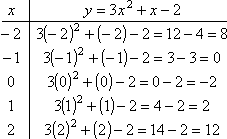Now I can do my graph, and I will label the vertex: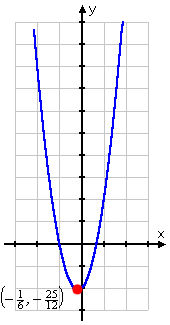When you write down the vertex in your homework, write down the exact coordinates: "( –1/6 , –25/12 )". But for graphing purposes, the decimal approximation of "(–0.2, –2.1)" may be more helpful, since it's easier to locate on the axes.

The only other consideration regarding the vertex is the "axis of symmetry". If you look at a parabola, you'll notice that you could draw a vertical line right up through the middle which would split the parabola into two mirrored halves. This vertical line, right through the vertex, is called the axis of symmetry. If you're asked for the axis, write down the line "x = h", where h is just the x-coordinate of the vertex. So in the example above, then the axis would be the vertical line x = h = –1/6.

Helpful note: If your quadratic's x-intercepts happen to be nice neat numbers (so they're relatively easy to work with), a shortcut for finding the axis of symmetry is to note that this vertical line is always exactly between the two x-intercepts. So you can just average the two intercepts to get the location of the axis of symmetry and the x-coordinate of the vertex. However, if you have messy x-intercepts (as in the example above) or if the quadratic doesn't actually cross the x-axis (as you'll see on the next page), then you'll need to use the formula to find the vertex.

<< Previous  Top  |  1 | 2 | 3 | 4  |  Return to Index  Next >>